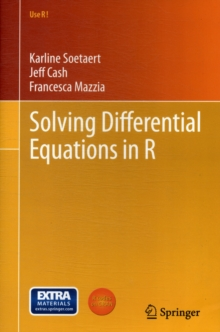Supporting your high street Find out how »
• My Account# Solving Differential Equations in R Paperback / softback

## Part of the Use R! series

#### Description

Mathematics plays an important role in many scientific and engineering disciplines.

This book deals with the numerical solution of differential equations, a very important branch of mathematics.

Our aim is to give a practical and theoretical account of how to solve a large variety of differential equations, comprising ordinary differential equations, initial value problems and boundary value problems, differential algebraic equations, partial differential equations and delay differential equations.

The solution of differential equations using R is the main focus of this book.

It is therefore intended for the practitioner, the student and the scientist, who wants to know how to use R for solving differential equations.

However, it has been our goal that non-mathematicians should at least understand the basics of the methods, while obtaining entrance into the relevant literature that provides more mathematical background.

Therefore, each chapter that deals with R examples is preceded by a chapter where the theory behind the numerical methods being used is introduced.

In the sections that deal with the use of R for solving differential equations, we have taken examples from a variety of disciplines, including biology, chemistry, physics, pharmacokinetics.

Many examples are well-known test examples, used frequently in the field of numerical analysis.

#### Information

• Format: Paperback / softback
• Pages: 248 pages, 10 Tables, black and white; 13 Illustrations, color; 50 Illustrations, black and white; X
• Publisher: Springer-Verlag Berlin and Heidelberg GmbH & Co. K
• Publication Date:
• Category: Differential calculus & equations
• ISBN: 9783642280696

#### Other Formats

£39.99

£33.15

on all orders

###### Pick up orders

from local bookshops

£74.99

£61.65

£64.99

£55.24

£40.00

£34.00